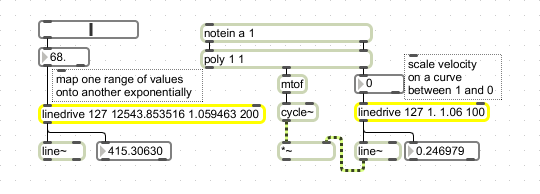# linedrive

Scale numbers exponentially

## Description

linedrive takes integers ranging from -[argument 1] to +[argument 1] and scales them to fall within the range -[argument 2] to +[argument 2]. If the input equals argument 1, the output is equal to argument value 2. Output varies exponentially with the input depending on argument 3.

## Arguments

Name Type Opt Description
maximum-input maximum-output scaling-curve int or float Obligatory. The first argument is the maximum input value, followed by the maximum output value. The third argument specifies the nature of the scaling curve. The third argument must be greater than 1. The larger the value, the more steeply exponential the curve is. An appropriate value for this argument is 1.06. The fourth argument is the initial delay time in milliseconds. This value can be changed via the right inlet.

## Messages

 int input-to-conversion [int] In left inlet: The number is converted according to the following expression y = b e^{-a log c} e^{x log c} where x is the input, y is the output, a, b, and c are the three typed-in arguments, and e is the base of the natural logarithm (approximately 2.718282). The output is a two-item list containing y followed by the delay time most recently received in the right inlet. (inlet1) delay-time [int] In right inlet: Sets the current delay time appended to the scaled output. A connected line~ object will ramp to the new target value over this time interval. float input-to-conversion [float] In left inlet: The number is converted according to the following expression y = b e^{-a log c} e^{x log c} where x is the input, y is the output, a, b, and c are the three typed-in arguments, and e is the base of the natural logarithm (approximately 2.718282). The output is a two-item list containing y followed by the delay time most recently received in the right inlet.

## Output

list: When an int or float is received in the left inlet, a list is sent out containing a scaled version of the input (see the formula above) and the current delay time.

## ExamplesUse linedrive for exponential value scaling